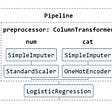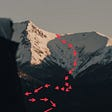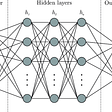Hi there! This article is part of a series called “Hello, <algorithm>”. In this series we will give some insights about how different AI algorithms work, and we will have fun by implementing them. Today we are gonna talk about Gradient Descent, a simple yet powerful optimization algorithm for finding the (local) minimum of a given function.

# Getting some insight

The inspiration behind Gradient Descent comes directly from calculus. Basically, it states that if we’ve a differentiable function, the fastest way to decrease is by taking steps proportional to the opposite direction of the function’s gradient at any given point. This happens because the gradient points to the steepest direction of the function’s generated surface at the current point.

In other words, think about the function’s surface as a mountain that you are hiking down. You know that your goal is to reach the bottom, and you may think that the fastest way to accomplish this is by proceeding through the path that makes you descend the most. In this case, that path points to the opposite of the steepest mountain direction upwards.

With this in mind, we can repeatedly perform these steps in the appropriate direction and we should eventually converge into the (local) minimum. Following our analogy, this is the equivalent of arriving to the bottom of our mountain.

# Calculating the next step

So we’ve been talking about taking steps in the right direction, but how can we calculate them? Well, the answer is in the following equation:

This formula needs some clarification. Let’s say we are currently in a position Θ⁰, and we want to get to a position Θ¹. As we said previously, every step is gonna be proportional to the opposite direction of the function’s gradient at any given point. So this definition of step for a given function J(Θ) will be equal to −α∇J(Θ).

## Why minus and not plus?

Remember that we take steps in the opposite direction of the gradient. So in order to achieve this, we subtract the step value to our current position.

## Okay that sounds good, but what do you mean with α?

The symbol α is called the Learning Rate. This is a value that will force us to take little steps so we don’t overshoot the (local) minimum. A bad choice for α would trap us into one of the following possibilities:

• If α is too small, our learning algorithm is gonna take too much time to converge.
• If α is too large, our learning algorithm might overshoot the bottom, and even diverge because of an infinite loop.

Take a look at the following examples to see what happens when we make a bad choice for the Learning Rate α:

The gradient of a function J(Θ) (denoted by ∇J(Θ)) is a vector of partial derivatives with respect to each dimension or parameter Θᵢ. Notational details are given in the equation below:

## A little example

To make this definition of gradient clearer, let’s calculate the gradient of the following function:

As we can see, this function contains three parameters or dimensions. Thus the appropriate way to proceed is by calculating the partial derivative with respect to each param:

Now we can group those values and that will give us the function’s gradient:

And that’s it! With this vector, we can get the steepest direction at any given point simply by replacing each parameter with its corresponding value:

# Hacking Time

And now, for the grand finale, we will go through a full example and we will code our own algorithm for gradient descent.

## Defining the example

In this section, we will apply linear regression in order to find the correct function approximation for a given set of points in a plane. The set of points we are trying to predict looks as follows:

As it’s common, the choice for J(Θ) will be the least-squares cost function for measuring the error of an approximation:

In the equation above:

• m is the amount of points in the set.
• ½ is a convenient constant that will cancel out when we take the gradient of J(Θ). This makes maths nicer and doesn’t affect the result.
• y is the real value of the y-coordinate for the ith point.
• h is our function approximation. It will give us the predicted y-coordinate for the ith-point using parameters Θ and input x.

Finally, before beginning to code let’s calculate the gradient vector of our function. You can see that as we’ve got two parameters for Θ, we will need to calculate two partial derivatives.

Okay, time to proceed. It’s important to mention that our implementation will be in a vectorized form. This means that we will transform all the formulas mentioned above into matrices operations. The advantages of this implementation are that code will be more concise, and with this our computer can take advantage of advanced underlying matrix algorithms.

To work with the vectorized form, we need to add a dummy variable x0 to each point with a value equal to 1. The reason for this is that when we perform matrix multiplication, the intercept parameter Θ0 will be multiplied with that 1 and it will maintain its value as the defined equations establishes.

Below you can see the vectorized form of the error function J(Θ) and its gradient ∇J(Θ):

## Coding time

With every function defined, we can proceed to code our algorithm. The first thing we should do is to declare the points dataset and the Learning Rate α.

Now we can proceed by defining the error function J(Θ) and its gradient ∇J(Θ). Remember everything will be defined in a vectorized way.

This is the heart of our code. Here we will perform steps that update Θ until we reach the (local) minimum. That is, when all the values of the gradient vector are less than or equal to some specified threshold (1/e⁵ in this case).

And we’re done! You can see the complete code in the snippet below:

Now we can run our algorithm and it will give us the optimal values for Θ that minimize the error. Below you can see the answers I obtained after running it on my computer:

This is the scatter plot we showed before with the line corresponding to the optimal Θ:

Well, we’ve finished our code and our article. I hope that you’d learned one thing or two about Gradient Descent, and more importantly, that you are now really excited about learning by taking a look at the further reading list.

--

--

--

## More from AI Society

Artificial Intelligence Hacking Group

## Back to the Machine Learning fundamentals: How to write Pipeline for Model deployment (Part 3/3)## Cloud Professional ML Engineer## Ray’s R&D Rundown: A NeurIPS Dive## Current state of voice cloning and how you can implement it yourself## NLP with Python and TextBlob## Universal Approximation Theorem: Code Refactoring for Software 2.0?## Modern Neural Networks (AlexNet) in the ILSVRC## Supervised Learning with R: Linear Regression## Using CNN to predict regressive STD drug efficacy score## The purpose of Activation functions in neural networks